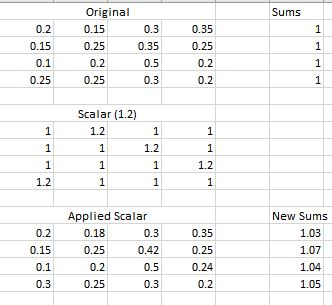## Applying Scalar, and Inverse Scalar

Hi,

I have a question regarding the capabilites of proc iml. Suppose I have a matrix of probabilites, then want to multiply each cell directly by a scalar matrix, so that only some of the probabilites go up while some remain the same. For example:Now, I'd like to multiply the other values in each row by a scalar in order for each row to still sum to one. But the scalar also needs to be computed for each row.

This can be accomplished with excel's solver, as shown:Would this be possible to accomplish in IML, or would I need to look to a different procedure, or is there a better way to go about this?

Attached is code to set up the beginning matrices in SAS:

``````proc iml;
A = {	0.20	0.15	0.30	0.35,
0.15	0.25	0.35	0.25,
0.10	0.20	0.50	0.20,
0.25	0.25	0.30	0.20};
B = A[ ,+];
C = {	1.0	1.2	1.0	1.0,
1	1	1.2	1.0,
1	1	1	1.2,
1.2	1	1	1};

D = A#C;
E = D[ ,+];

``````

Thank you,

Justin

1 ACCEPTED SOLUTION

Accepted Solutions

## Re: Applying Scalar, and Inverse Scalar

The problem is that (H ^= 0) first evaluates to a matrix of zeros and ones, so when this is applied to D with elementwise multiplication it either preserves the existing values in D, or sets them to zero.

It is possible to get the Inverse Scalar Matrix using a small trick. First subtract one from G, next calculate H as you have above, finally add back the one.  The overall effect is to place a one, rather than a zero, at every location in H corresponding to one of the modified values.

``````F = (D#(C^=1))[,+];
G = (1-F)/(E-F);

H = (G-1)#(C=1) + 1;
J = D # H;
print H [l='Inverse Scalar Matrix'], J [l='Finished Matrix'];``````
4 REPLIES 4

## Re: Applying Scalar, and Inverse Scalar

I think you are over complicating things by suggesting you need to use the Excel solver.  Once you know the sum of values in each row that have been increased (F), then it is a simple calculation to find the row scalars (G).

``````F = (D#(C^=1))[,+];
G = (1-F)/(E-F);
print G;``````

## Re: Applying Scalar, and Inverse Scalar

That works great; thank you.

Now to apply that to the new matrix, so that the values of G multiply all the other cells in D,  I tried:

``````	H = G#(C=1);  /*To create similar scalar matrix */
J = D#(H ^= 0);

``````

But J isn't showing the values I'd like.

## Re: Applying Scalar, and Inverse Scalar

The problem is that (H ^= 0) first evaluates to a matrix of zeros and ones, so when this is applied to D with elementwise multiplication it either preserves the existing values in D, or sets them to zero.

It is possible to get the Inverse Scalar Matrix using a small trick. First subtract one from G, next calculate H as you have above, finally add back the one.  The overall effect is to place a one, rather than a zero, at every location in H corresponding to one of the modified values.

``````F = (D#(C^=1))[,+];
G = (1-F)/(E-F);

H = (G-1)#(C=1) + 1;
J = D # H;
print H [l='Inverse Scalar Matrix'], J [l='Finished Matrix'];``````Ksharp
Super User

## Re: Applying Scalar, and Inverse Scalar

It looks like you want SOLVE() function.

``````
proc iml;
A = {	0.20	0.15	0.30	0.35,
0.15	0.25	0.35	0.25,
0.10	0.20	0.50	0.20,
0.25	0.25	0.30	0.20};

C = {	1.0	1.2	1.0	1.0,
1	1	1.2	1.0,
1	1	1	1.2,
1.2	1	1	1};

D=A#C;
Y=j(nrow(A),1,1);
X=solve(D,Y);
want=D#X`;
print want (want[,+]);
quit;``````

WANT

0.2467064 0.0667512 0.3381478 0.3483947   1
0.1850298 0.0927101 0.4734069 0.2488533   1
0.1233532 0.074168 0.5635796 0.2388992     1
0.3700595 0.0927101 0.3381478 0.1990827   1

From The DO Loop